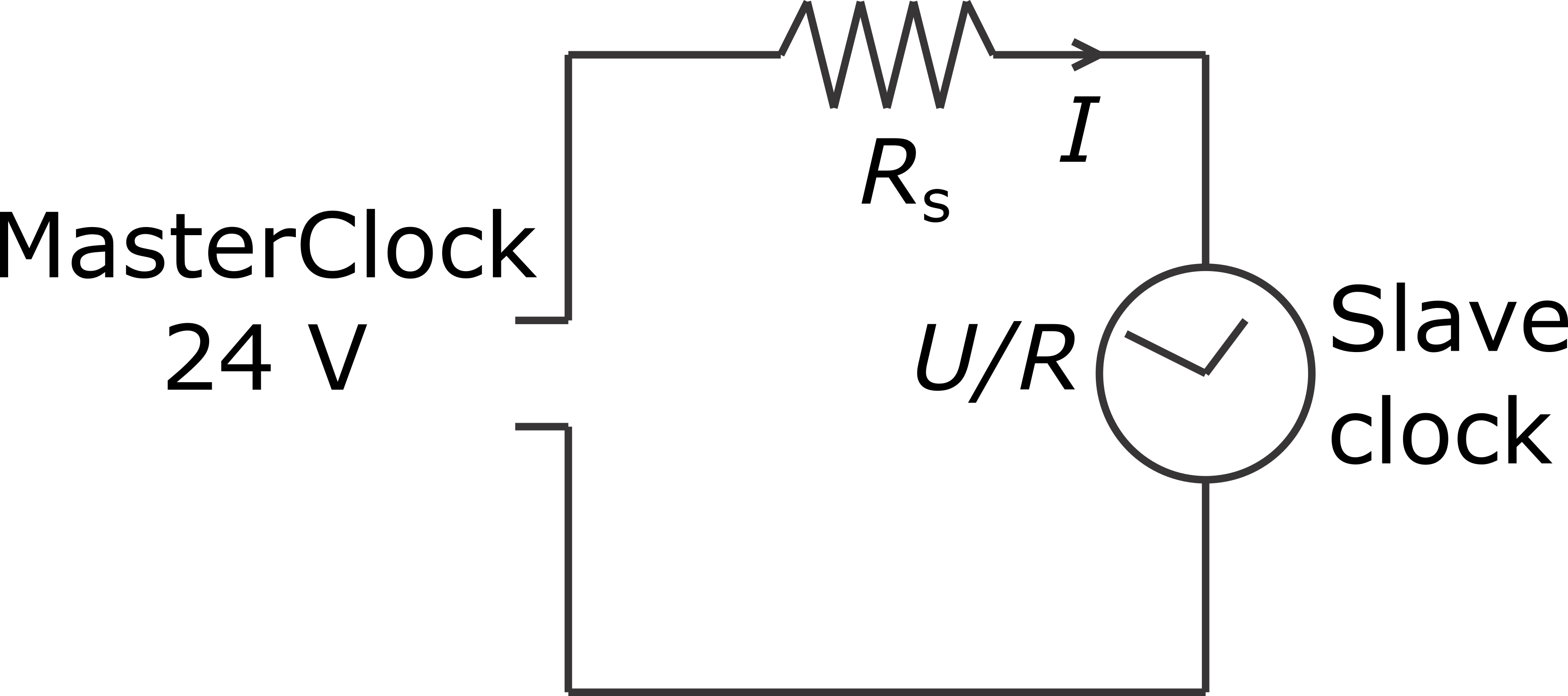﻿ Series resisor calculator If you have a slave clock which requires a different voltage than 24 volts, you can add a resistor in series with the master clock device to decrease the voltage for your slave clock. Use the calculator below to calculate the value for the resistor Rs. To calculate the value for the series resistor, either the required current or coil resistance of the slave clock is needed. Typically you can find the required voltage and current/resistance values for your slave clock behind your clock, near the mechnanism.Required voltage (U): V
Required current (I): mA or Measured resistance (R): ohms

Resistor value (Rs): ohms